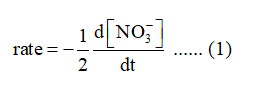# A:Consider the following reaction:4 Zn(s) + 10 H+ + 2 NO3-  -->  4 Zn2+ + N2O(g) + 5 H2O(l)If the rate of NO3- disappearance 8.4 x 10-4 M/s, what is the overall reaction rate?Group of answer choices4.2 x 10-4 M/s4.2 x 10-3 M/s8.4 x 10-4 M/s1.7 x 10-3 M/s B:Consider the following reaction:4 Zn(s) + 10 H+ + 2 NO3-  -->  4 Zn2+ + N2O(g) + 5 H2O(l)What is the rate law for this reaction?Group of answer choicesrate = k [H+][NO3-]rate = k [H+]10[NO3-]2[Zn2+]4[N2O]More information is needed to determine this.rate = k [H+]10[NO3-]2

Question
54 views

A:

Consider the following reaction:

4 Zn(s) + 10 H+ + 2 NO3-  -->  4 Zn2+ + N2O(g) + 5 H2O(l)

If the rate of NO3- disappearance 8.4 x 10-4 M/s, what is the overall reaction rate?

4.2 x 10-4 M/s

4.2 x 10-3 M/s

8.4 x 10-4 M/s

1.7 x 10-3 M/s

B:

Consider the following reaction:

4 Zn(s) + 10 H+ + 2 NO3-  -->  4 Zn2+ + N2O(g) + 5 H2O(l)

What is the rate law for this reaction?

rate = k [H+][NO3-]

rate = k [H+]10[NO3-]2[Zn2+]4[N2O]

rate = k [H+]10[NO3-]2

check_circle

Step 1

Hello. Since you have posted multiple questions and not specified which question needs to be solved, we will solve the first question for you. If you want any other specific question to be solved then please resubmit only that question or specify the question number.

Step 2

According to the differential rate law, the rate of reaction with respect to the rate of disappearance of nitrate ion is as follows:...

### Want to see the full answer?

See Solution

#### Want to see this answer and more?

Solutions are written by subject experts who are available 24/7. Questions are typically answered within 1 hour.*

See Solution
*Response times may vary by subject and question.
Tagged in

### Chemistry